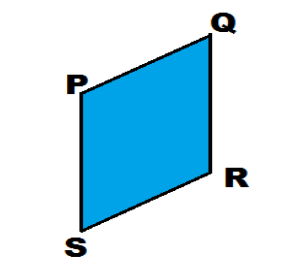In the diagram, points P And Q are the vertices of a quadrilateral PQRS. Given that the coordinates of R and S are $(8,0)$ and $(4, - 2)$ respectively, the figure PQRS is likely to be :(a) Parallelogram (b) Rhombus (c) Trapezium (d) KiteVerified
149.4k+ views
Hint: In this question try to plot the R and S point on the graph and observe the graph carefully. A parallelogram is the quadrilateral in which opposite sides are parallel. If all the sides of the parallelogram are equal then it becomes the Rhombus. In the trapezium the two sides are parallel.

By observing the figure we will find out that the point P $(4,2)$ and the point Q is $(8,4)$ .
And it is given in the question that the points R and S are $(8,0)$ and $(4, - 2)$ respectively.So by observing the point P and S have the same X - Coordinate and the variation in Y coordinate is $4$. Hence the length of Line PS is $4unit$.
Similarly for the Point Q and R both have the same X- Coordinate the variation in Y coordinate is $4$. Hence the length of Line QR is $4unit$.
And by observing the figure the line PS and QR are parallel to each other .
Now we have to calculate the length of PQ and SR.
By the distance formula length of PQ , the point P $(4,2)$ and the point Q is $(8,4)$
$= \sqrt {{{\left( {{x_2} - {x_1}} \right)}^2} + {{({y_2} - {y_1})}^2}}$
= $\sqrt {{{\left( {4 - 8} \right)}^2} + {{(2 - 4)}^2}}$
$= \sqrt {16 + 4}$
PQ = $2\sqrt 5$
Similarly
By the distance formula length of RS , the point R and S are $(8,0)$ and $(4, - 2)$ respectively.
$= \sqrt {{{\left( {{x_2} - {x_1}} \right)}^2} + {{({y_2} - {y_1})}^2}}$
= $\sqrt {{{(8 - 4)}^2} + {{(0 + 2)}^2}}$
$= \sqrt {16 + 4}$
RS = $2\sqrt 5$
Hence the length PQ and RS are equal . By observing the graph we will see that the line RS and PQ is parallel .
So the PQ and RS are equal and parallel to each other .
And the line PS and QR are parallel and equal to each other .
So quadrilaterals in which opposite sides are parallel and equal are called parallelograms .
So the answer is Parallelogram. Hence option “A” will be correct .

Note: In Parallelogram the sum of adjacent angles is ${180^\circ }$.
Kite and Its Properties
Two pairs of sides known as consecutive sides are equal in length. One pair of diagonally opposite angles is equal in measurement. The diagonals meet each other at ${90^\circ}$ , this means that they form a perpendicular bisector.